## 2.7  Euler Angles

To go further we must finally specify a set of generalized coordinates. We first do this using the traditional Euler angles. Later, we find other ways of describing the orientation of a rigid body.

We are using an intermediate representation of the orientation in terms of the function M of the generalized coordinates that gives the rotation that takes the body from some reference orientation and rotates it to the orientation specified by the generalized coordinates. Here we take the reference orientation so that principal-axis unit vectors hata,,are coincident with the basis vectorsi, labeled here by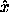,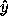,.

We define the Euler angles in terms of simple rotations about the coordinate axes. Let Rx(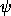) be a right-handed rotation about theaxis by the angle, and let Rz() be a right-handed rotation about theaxis by the angle. The function M for Euler angles is written as a composition of three of these simple coordinate axis rotations: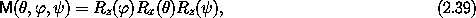for the Euler angles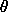,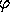,.

The Euler angles can specify any orientation of the body, but the orientation does not always correspond to a unique set of Euler angles. In particular, if= 0 then the orientation is dependent only on the sum+, so the orientation does not uniquely determine eitheror.

Exercise 2.8.  Euler angles
It is not immediately obvious that all orientations can be represented in terms of the Euler angles. To show that the Euler angles are adequate to represent all orientations, solve for the Euler angles that give an arbitrary rotation R. Keep in mind that some orientations do not correspond to a unique representation in terms of Euler angles.

Though the Euler angles allow us to specify all orientations and thus can be used as generalized coordinates, the definition of Euler angles is pretty arbitrary. In fact no reasoning has led us to them. This is reflected in our presentation of them by just saying ``here they are.'' Euler angles are well suited for some problems, but cumbersome for others.

There are other ways of defining similar sets of angles. For instance, we could also take our generalized coordinates to satisfy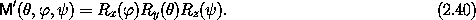Such alternatives to the Euler angles come in handy from time to time.

Each of the fundamental rotations can be represented as a matrix. The rotation matrix representing a right-handed rotation about theaxis by the angleis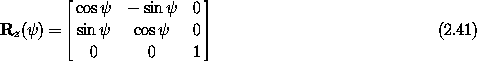and a right-handed rotation about the x axis by the angleis represented by the matrix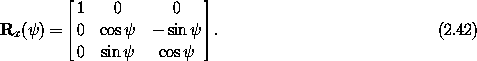The matrix that represents the rotation that carries the body from its reference orientation to the actual orientation is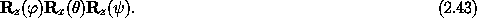The rotation matrices and their product can be constructed by simple programs:

(define (rotate-z-matrix angle)
(matrix-by-rows
(list (cos angle) (- (sin angle))               0)
(list (sin angle)     (cos angle)               0)
(list           0               0               1)))

(define (rotate-x-matrix angle)
(matrix-by-rows
(list           1               0               0)
(list           0     (cos angle) (- (sin angle)))
(list           0     (sin angle)     (cos angle))))

(define (Euler->M angles)
(let ((theta (ref angles 0))
(phi   (ref angles 1))
(psi   (ref angles 2)))
(* (rotate-z-matrix phi)
(rotate-x-matrix theta)
(rotate-z-matrix psi))))

Now that we have a procedure that implements a sample M, we can find the components of the angular velocity vector and the body components of the angular velocity vector using the procedures M-of-q->omega-of-t and M-of-q->omega-body-of-t from section 2.6. For example,

(show-expression
(((M-of-q->omega-body-of-t Euler->M)
(up (literal-function 'theta)
(literal-function 'phi)
(literal-function 'psi)))
't))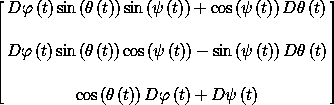To construct the kinetic energy we need the procedure of state that gives the body components of the angular velocity vector:

(show-expression
((M->omega-body Euler->M)
(up 't
(up 'theta 'phi 'psi)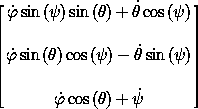We capture this result as a procedure:

(define (Euler-state->omega-body local)
(let ((q (coordinate local)) (qdot (velocity local)))
(let ((theta (ref q 0))
(psi (ref q 2))
(phidot (ref qdot 1))
(psidot (ref qdot 2)))
(let ((omega-a (+ (* thetadot (cos psi))
(* phidot (sin theta) (sin psi))))
(omega-b (+ (* -1 thetadot (sin psi))
(* phidot (sin theta) (cos psi))))
(omega-c (+ (* phidot (cos theta)) psidot)))
(column-matrix omega-a omega-b omega-c)))))

The kinetic energy can be written:

(define ((T-rigid-body A B C) local)
(let ((omega-body (Euler-state->omega-body local)))
(* 1/2
(+ (* A (square (ref omega-body 0)))
(* B (square (ref omega-body 1)))
(* C (square (ref omega-body 2)))))))